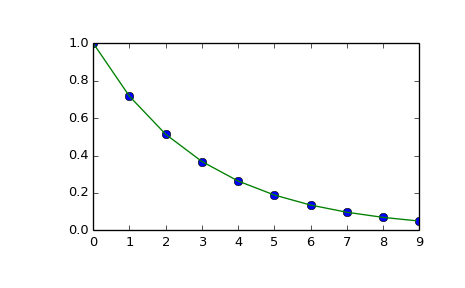# scipy.interpolate.interp1d¶

class scipy.interpolate.interp1d(x, y, kind='linear', axis=-1, copy=True, bounds_error=True, fill_value=nan, assume_sorted=False)[source]

Interpolate a 1-D function.

x and y are arrays of values used to approximate some function f: y = f(x). This class returns a function whose call method uses interpolation to find the value of new points.

Parameters: x : (N,) array_like A 1-D array of real values. y : (...,N,...) array_like A N-D array of real values. The length of y along the interpolation axis must be equal to the length of x. kind : str or int, optional Specifies the kind of interpolation as a string (‘linear’, ‘nearest’, ‘zero’, ‘slinear’, ‘quadratic, ‘cubic’ where ‘slinear’, ‘quadratic’ and ‘cubic’ refer to a spline interpolation of first, second or third order) or as an integer specifying the order of the spline interpolator to use. Default is ‘linear’. axis : int, optional Specifies the axis of y along which to interpolate. Interpolation defaults to the last axis of y. copy : bool, optional If True, the class makes internal copies of x and y. If False, references to x and y are used. The default is to copy. bounds_error : bool, optional If True, a ValueError is raised any time interpolation is attempted on a value outside of the range of x (where extrapolation is necessary). If False, out of bounds values are assigned fill_value. By default, an error is raised. fill_value : float, optional If provided, then this value will be used to fill in for requested points outside of the data range. If not provided, then the default is NaN. assume_sorted : bool, optional If False, values of x can be in any order and they are sorted first. If True, x has to be an array of monotonically increasing values.

UnivariateSpline
An object-oriented wrapper of the FITPACK routines.
interp2d
2-D interpolation

Examples

>>> import matplotlib.pyplot as plt
>>> from scipy import interpolate
>>> x = np.arange(0, 10)
>>> y = np.exp(-x/3.0)
>>> f = interpolate.interp1d(x, y)

>>> xnew = np.arange(0, 9, 0.1)
>>> ynew = f(xnew)   # use interpolation function returned by interp1d
>>> plt.plot(x, y, 'o', xnew, ynew, '-')
>>> plt.show()Methods

 __call__(x) Evaluate the interpolant :Parameters: x : array_like Points to evaluate the interpolant at.

#### Previous topic

scipy.interpolate.approximate_taylor_polynomial

#### Next topic

scipy.interpolate.interp1d.__call__